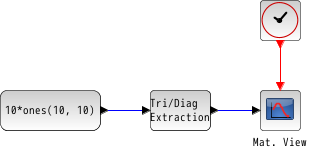Scilab Home page | Wiki | Bug tracker | Forge | Mailing list archives | ATOMS | File exchange
Change language to: English - Français - Português - Русский
Scilabヘルプ >> Xcos > palettes > Matrix operation palette > EXTTRI

EXTTRI

Triangular or Diagonal Extraction

Block ScreenshotDescription

The EXTTRI block extracts some elements from the input matrix u. The size of the output matrix is the same that those of the input matrix.

When the extraction type parameter is set to:

• 1 (Upper triangle): the block copies the elements on and above the main diagonal to an output matrix. The elements below the main diagonal are set to zero.

• 2 (Lower triangle): the block copies the elements on and below the main diagonal to an output matrix. The elements above the main diagonal are set to zero.

• 3 (Diagonal): the block copies the elements on the main diagonal to an output matrix. The elements above and below the main diagonal are set to zero.

Equivalent functions of EXTTRI in Scilab are triu(u), tril(u) and diag(u), respectively

Parameters• Datatype(1=real double 2=Complex)

It indicates the type of the output. It support only the two types double (1) and complex (2). If we input another entry in this label xcos will print the message "Datatype is not supported".

Properties : Type 'vec' of size 1.

• Extraction type (1=lower 2=upper 3=diagonal)

It indicates the form of the output matrix. It can be an upper triangle, a lower triangle or a diagonal matrix.

Properties : Type 'vec' of size 1.

Default properties

• always active: no

• direct-feedthrough: yes

• zero-crossing: no

• mode: no

• regular inputs:

- port 1 : size [-1,-2] / type 1

• regular outputs:

- port 1 : size [-1,-2] / type 1

• number/sizes of activation inputs: 0

• number/sizes of activation outputs: 0

• continuous-time state: no

• discrete-time state: no

• object discrete-time state: no

• name of computational function: extrilz

Example

A = [1 2 3 ; 4 5 6 ; 7 8 9 ; 10 11 12]
If the extraction type is 2 then the output is
B = [1 0 0 ; 4 5 0 ; 7 8 9 ; 10 11 12]

Example of diagonal extractionInterfacing function

• SCI/modules/scicos_blocks/macros/MatrixOp/EXTTRI.sci

Computational function

• SCI/modules/scicos_blocks/src/c/exttril.c
• SCI/modules/scicos_blocks/src/c/exttriu.c
• SCI/modules/scicos_blocks/src/c/extdiag.c
• SCI/modules/scicos_blocks/src/c/exttrilz.c
• SCI/modules/scicos_blocks/src/c/exttriuz.c
• SCI/modules/scicos_blocks/src/c/extdiagz.c

• EXTRACT — Matrix extractor
• triu — 上三角
• tril — 行列の下三角部
• diag — 対角行列の構築または展開